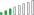cancel
Showing results for
Did you mean:

Fabric is Generally Available. Browse Fabric Presentations. Work towards your Fabric certification with the Cloud Skills Challenge.Helper I

## Use measure in calculated row for multiple rows

I have a table which looks like this:

 Value: AbsValue: InLast7Days: IsSignificant: 500 500 1 1 -600 600 1 1 -8,000 8,000 0 0 25,000 25,000 0 0 40 40 1 0

I would like to make a column where we indicate whether or not the value is significant. This is based on the standard deviation formula:

StDev:= CALCULATE( STDEV.P ( Value ) , InLast7Days = 1 ) = 451

In the IsSignificant I would like a flag to mark 1 if the AbsValue is larger than the standard deviation just calculated, and 0 if it is not larger.

I've tried using the StDev measure in the calculated column IsSignificant, but then they all equal 1, and if I try and make the calculation within the calculated column instead, only the ones that are InPast7Days are 1 regardless of their value.

I've found that the reason for this, is because if you just write the measure calculation as the calculated column, then they all equal 0, it seems that it is just calculating the standard deviation for the one row, but I need the 'total' measure in each individual row.

Hope this makes sense, and that someone has an answer that can help me 🙂

1 ACCEPTED SOLUTIONResolver III

hope this helps.

When performing the STD calculation you need to tell CALCULATE which context you want to consider.

The context you described is all rows where the last seven days flag is one.

It can be a bit of a mind bender, but as grasped is amazingly powerful.

``````=var _STD=CALCULATE(STDEV.P(Table1[Value]),
filter(ALL(Table1),[InLast7Days]=1)
)
return if(Table1[AbsValue]>_STD &&
Table1[InLast7Days]=1,
1,0)``````2 REPLIES 2Resolver III

hope this helps.

When performing the STD calculation you need to tell CALCULATE which context you want to consider.

The context you described is all rows where the last seven days flag is one.

It can be a bit of a mind bender, but as grasped is amazingly powerful.

``````=var _STD=CALCULATE(STDEV.P(Table1[Value]),
filter(ALL(Table1),[InLast7Days]=1)
)
return if(Table1[AbsValue]>_STD &&
Table1[InLast7Days]=1,
1,0)``````Helper I

This works so well, thank you so much!
If i would like to add an additional filter to the _STD calculation, like an EstimateType which could be 1, 2 or 3, could I just add it like this?

``````=var _STD=CALCULATE(STDEV.P(Table1[Value]),
filter(ALL(Table1),[InLast7Days]=1)
, [EstimateType] = 2)``````

Or would I have to add it in a different way?Announcements#### Power BI Monthly Update - November 2023

Check out the November 2023 Power BI update to learn about new features.#### Fabric Community News unified experience

Read the latest Fabric Community announcements, including updates on Power BI, Synapse, Data Factory and Data Activator.#### The largest Power BI and Fabric virtual conference

130+ sessions, 130+ speakers, Product managers, MVPs, and experts. All about Power BI and Fabric. Attend online or watch the recordings.Top Solution Authors
Top Kudoed Authors
Users online (1,767)